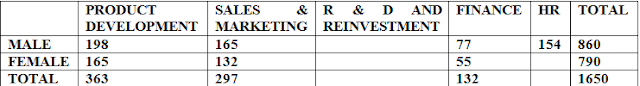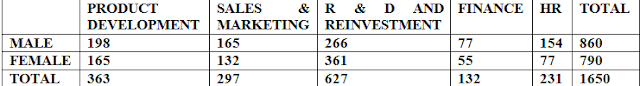# Tricks To Solve Caselet DI With Example### Introduction

Caselet DI is trending these days and whenever anything starts trending, we must know about that.
Caselet DI is not new or not even out of way. It is just a mathematical form of English Comprehension. In Caselet DI, a long paragraph is given and on the basis of that, some questions are asked. Isn’t it English Comprehension?
• In a given paragraph of Caselet DI, lots of information is given.
• You have to read the paragraph carefully and then you have to note down all the key information as short as possible.
• The given information will let you draw some diagrams such as Venn diagram, tabular chart or any other diagram.
• The difference between simple DI and Caselet DI is - In simple DI, information is already given in diagrammatical forms but in Caselet DI, you have to draw a diagram on the basis of given information.
Before start solving Caselet DI, you must have knowledge of following things.
• How to draw a diagram on the basis of given information.
• Which diagram is the need of the question?
• Knowledge of Venn diagram.
• Knowledge of the relationship between fractions and their percentage forms.
• Knowledge of simplification and approximation.
• If the question belongs to CI & SI or Profit & Loss or Speed, time and distance then you must have knowledge of basic formulae of these topics.
• Must have knowledge of Ratio and Proportion.

To make few things more clear, let us take a simple question:
In a college, some students like English, some like Maths and some like Science. Some like both English and Maths but not Science, some like both Maths and Science but not English and Some like both Science and English but not Maths. Some like all three subjects.

The given information can be simply put in a Venn diagram as follow:• A, B and C are representing the whole circle.
• A means some like English, B means some like Maths and C means some like Science.
• Region D means some like both English and Maths but not Science, region E means some like both Maths and Science but not English and region F means some like both Science and English but not Maths.
• Region G means some like all these three subjects.

After arranging the information in Venn diagram, we conclude following more information:
• Students who like only English= A- (D+F+G).
• Students who like only Maths= B-(D+E+G).
• Students who like only Science= C-(E+F+G).
NOTE: Practice more problems so that you may know the different forms of Caselet DI. While solving, must follow the above steps.

## A Caselet Question Asked In BOB PO Exam, 2017

A total of 1650 employees is working in a company in different departments. The ratio of male employees to female employees in the organisation is 86:79. There are total 5 departments in the company i.e. Product Development, Sales and Marketing, R & D and Reinvestment, Finance and HR. Total 198 males work in Product Development department. 18% employees work in Sales and Marketing department in which male to female ratio is 5:4. In Finance Department, 77 males are working and the number of females in this department is 5/7th of the number of males. The number of males in Sales and Marketing department is equal to the number of females in Product Development department. The number of males in Finance department is half of the number of males in HR department. Male to female ratio in R & D and Reinvestment department is 14:19.

1. The number of males in R & D and Reinvestment department is how much more than females in Product Development?
2. Female in R & D and Reinvestment department is what % of the total number of females in the company (approximately)?
3. The number of females in Finance department is what percent less than the number of females in Product Development department?

### SOLUTION:

#### Step 2

• Note down the given data and make calculation as per as the requirement
• TOTAL=1650
• M:F=86:79 then we can find the number of males and females as follows:
• 86+79=165= 1650,
• 1=10,
• 86=860 (total number of male),
• 79=790 (total number of female).
• Males in Product Development department= 198
• 18% employees work in Sales and Marketing department i.e. (18/100)*1650=297;
• Male to female ratio is 5:4 in the Sales and Marketing department, so 5+4=9=297.
• Therefore, number of male in this department=5*33=165 and the number of female in this department=4*33=132.
• Number of Male in Finance department=77 and female in this department is 5/7th of male. So number of female in this department= (5/7)*77=55.
• Number of males in Sales and Marketing department is equal to the number of females in Product Development department. So number the number of female in Product Development= 165.
• Number of males in Finance department is half of the number of males in HR department. So the number of male in HR department=2*77=154.
• Male to female ratio in R & D and Reinvestment department is 14:19.

#### Step 3

Now put these data in the table.'• Number of males in R & D and REINVESTMENT= 860-(198+165+77+154) = 266.
• 14=266 so 19=19*19=361 (Number of female in R & D and REINVESTMENT).
• Number of female in HR department= 790-(165+132+361+55)=77.

#### Step 4

Now put these data in remaining blanks in the table:#### Step 5

1) Male in R & D and Reinvestment=266.
Female in Product Development=165.
So, the number of males in R & D and Reinvestment department is more than females in Product Development= 266-165=101.

2) Female in R & D and Reinvestment=361.
The total number of females=790. So, (361/790)*100=45.7%.

3) Female in Finance Department=55.
Female in Product Department=165.
So, {(165-55)/165}*100= (1/3) * 200= 33(1/3)% *2=66(2/3)% (use the concept of percentage and fraction)